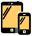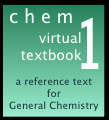# chem1 virtual textbook

## beginner / First Semester

1   What is Chemistry all about, anyway?
Chemistry in a nutshell: The major concepts and ideas of chemical science, and a look at some of the major currents of modern Chemistry. We hope this will motivate you get through the less-fun stuff in the first-year course!
2  Getting started in Chemistry: essential background
Classification and properties of matter • density and buoyancy • energy, heat and temperature • units and dimensions • measurement error and uncertainty • significant figures and rounding off
3  The basics of atoms, moles, formulas, equations
These five units take you through basic atomic theory and chemical arithmetic, and how chemicals are named. You will need these skills in amost everything that comes later.
4  What is pseudoscience?
How to tell the difference from science. Pseudoscience, junk science and quackery are pervasive in our culture, and all-too-many science courses simply ignore it.

## First-semester general chemistry5a  Primer on quantum theory of the atom
A quantum catechism: elementary introduction to quantum theory in the form of a question-and-answer "primer", emphasizing the concepts with a minimum of mathematics.

5b  Atomic structure and the periodic table
Everything you need to know in a first-year college course about the principal concepts of quantum theory as applied to the atom, and how this determines the organization of the periodic table.
5c  Why doesn't the electron fall into the nucleusOpposite charges attract, so why not? It's surprising how few textbooks explain this properly!

Properties of gases: matter at its simplest

A six-part treatment of the gaseous state of matter. Includes numerous examples of application of kinetic molecular theory and a section on real gases.
7  Solids and liquids
7a  States of matter
condensed states, liquids, types of solids, intermolecular forces, types of molecular units; hydrogen bonding and water; introduction to crystals, ionic solids, cubic and close-packed lattices. Liquids and interfacial effects. Changes of state: vapor pressure, boiling, and phase maps. Polymers and plastics.

7b  Solutions: their chemistry and physical properties
types of solutions, expressing concentrations, colligative properties and Raoult's law, solutions of volatile substances, osmosis, distillation. Solubility and solubility products, competing equilibria, important solubility systems. Electrolytes: nature of dissolved ions, electrolytic conductance, ionic migration, ground conduction.
8  Chemical bonding and molecular structure
Ten-part tutorial set on covalent bonding and polar covalence, shapes of molecules (VSEPR theory), hybrid orbitals, molecular orbitals applied to simple diatomics, introduction to transition metal d-orbital splitting and band theory of metals and semiconductors.
9 Understanding acids and bases
Covers the fundamental concepts of acids and bases. Except for some stoichiometry and a discussion on pH, this section is largely qualitative. Acid-base equilibrium calculations are covered in Unit 21.

This thorough treatment sets out the underlying concepts without invoking thermodynamics or complicated calculations; considerable emphasis is placed on the distinction between Q and K. The section on equilibrium calculations contains problem examples illustrating techniques such as iterative and graphical solutions of polynomials, all of which employ the "systematic" method of organizing information.
21  Acid-base equilibria and calculations

Acid-base chemistry can be extremely confusing, particularly when dealing with weak acids and bases. This set of lessons presents an updated view of the Brønsted-Lowry theory that makes it easy to understand answers to common questions: What's the fundamental difference between a strong acid and a weak acid? Can acid A neutralize base B? Why are some salts acidic and others alkaline? How do buffers work? What governs the shapes of titration curves?

This complete rewrite of the previous 1996 pdf document is now organized into seven lessons covering all aspects of the subject. Considerable effort has been expended to make most of this material accessible to those whose knowledge of thermodynamics is minimal, while still incorporating the proton-free energy concept that makes it easy to answer the questions posed in the preceding paragraph.

Much emphasis is placed on the practical aspects of calculations, including how to deal with quadratic equations and how to judge when approximations are appropriate. The commonly-taught algebraic method of solving acid-base problems hides the underlying principles and is able to deal with only the simplest systems. The use of log-C vs pH plots avoids the math, provides a bird's-eye view of what's going on in the solution, and yield useful results without algebra. Finally, there are separate lessons on practical applications (physiology, acid rain, and the geochemical carbonate system) and, for more advanced students, on exact numerical solutions.
22 Chemical Energetics
Introduction to thermodynamics • the First Law • enthalpy • molecules as energy carriers and converters • thermochemistry and calorimetry • some applications of enthalpy and the First Law
23  Thermodynamics of chemical equilibrium
All about entropy, free energy, and why chemical reactions do or don't take place. Energy spreading and spontaneous change • What is entropy? • The Second Law • Gibbs free energy • free energy and equilibrium • some applications of entropy and free energy
24  All about electrochemistry New version 2018
Chemistry and electricity • galvanic cells and electrodes • potential differences at interfaces • cell potentials and thermodynamics • Nernst equation and its applications • batteries and fuel cells • electrochemical corrosion • electrolytic cells and electrolysis
26 Chemical kinetics and reaction mechanisms
Rate of a reaction, rate laws • integrated rate laws, half-life• collision theory, activation energy and the Arrhenius equation • elementary processes, reaction mechanisms and chain reactions • kinetics of reactions in solution • catalysts and catalysis • experimental methods

30 The measure of matter
The first three sections of this unit cover units and dimensions, measurement error and significant figures and are duplicated in an early first-semester unit. The last two sections, reliability of a measurement and drawing conclusions from data introduce simple statistics that are needed in analytical chemistry courses.
31 The fall of the electron (oxidation-reduction)
How to predict the directions of oxidation-reduction reactions while avoiding formal electrochemistry. Similar to Fall of the Proton unit 21c. Coverage of biological redox reactions makes this a useful supplement for biochemistry courses.

33  Understanding entropyIn contrast to the common but misleading "entropy is disorganization" line, this tutorial describes entropy as a measure of the spreading and sharing of thermal energy. This concept provides rational, non-mathematical explanations of the effects of temperature change on reaction equilibria and on the colligative properties of solutions.Stephen Lower is a retired faculty member of the Dept of Chemistry, Simon Fraser UniversityBelow: engraving by Bernard Picart (1673-1733) for an illustration to Fontenelle's Oevres Diverses (1729) depicting an alchemy laboratory
with alembic at centre, and, at right, four putti gathered around a crucible; at left, putto carrying coal in a basketThis is the Main menu for the Chem1 Virtual Textbook, a free, online reference textbook for General Chemistry by of Simon Fraser University .

The Chem1 Virtual Textbook is a free resource aimed mainly at the first-year university level. It offers a more comprehensive, organized, and measured approach than is found in most standard textbooks. Although directed mainly at the first-year college level,is is also helpful as review material for students in more advanced courses in chemistry, and in biology, geology, and engineering.